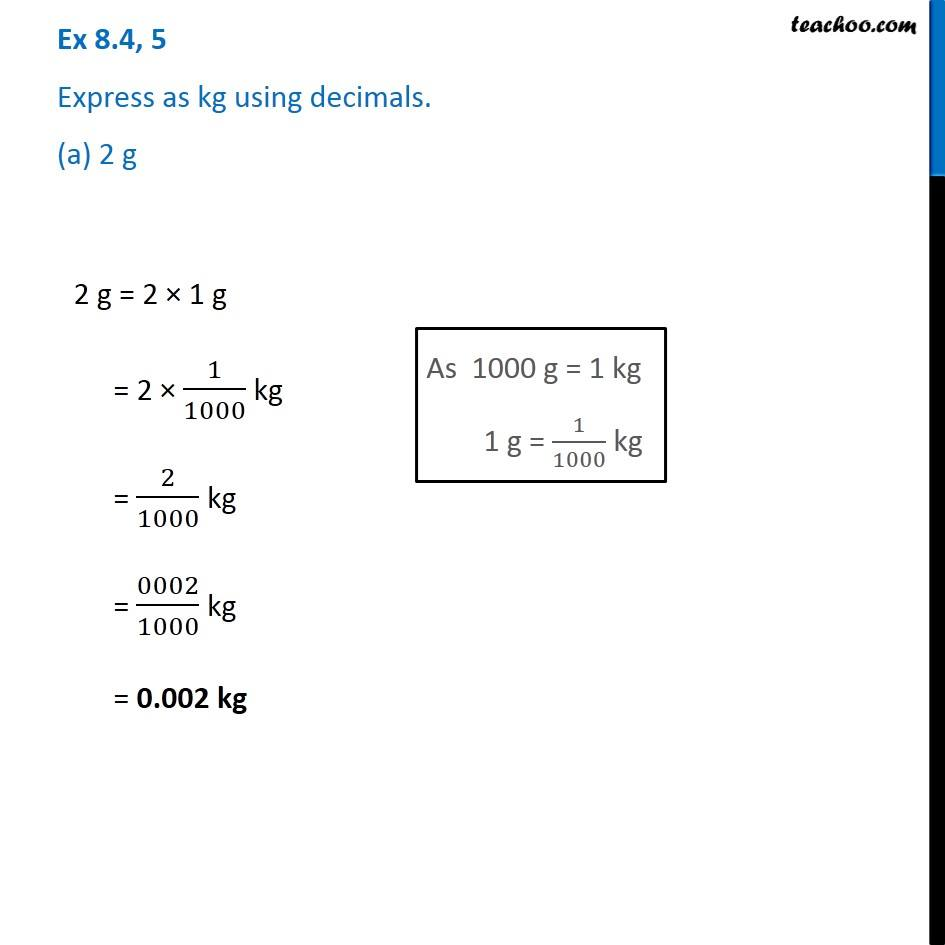Using Decimals - Converting to mm, cm, km, kg, rupees

Chapter 8 Class 6 Decimals
Concept wise### Transcript

Ex 8.4, 5 Express as kg using decimals. (a) 2 g 2 g = 2 × 1 g = 2 × 1/1000 kg = 2/1000 kg = 0002/1000 kg = 0.002 kg